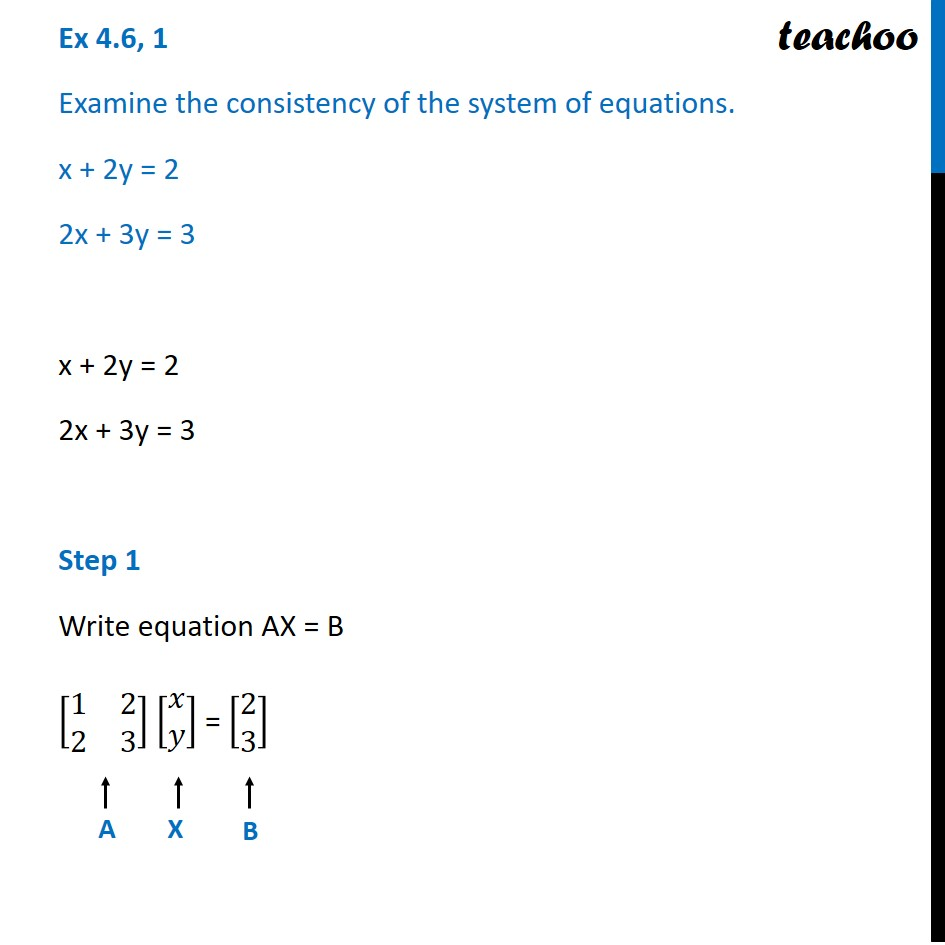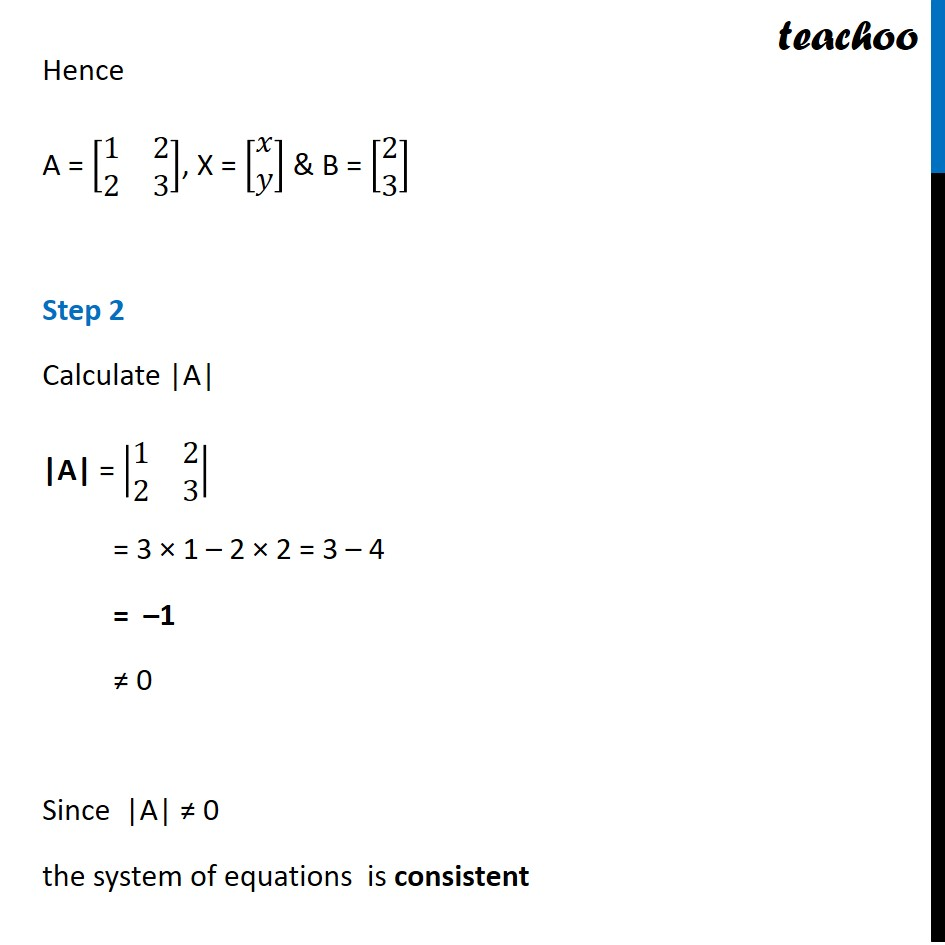Ex 4.5

Chapter 4 Class 12 Determinants
Serial order wiseLearn in your speed, with individual attention - Teachoo Maths 1-on-1 Class

### Transcript

Ex 4.5, 1 Examine the consistency of the system of equations. x + 2y = 2 2x + 3y = 3 x + 2y = 2 2x + 3y = 3 Step 1 Write equation AX = B [■8(1&[email protected]&3)] [■8(𝑥@𝑦)] = [■8([email protected])] Hence A = [■8(1&[email protected]&3)], X = [■8(𝑥@𝑦)] & B = [■8([email protected])] Step 2 Calculate |A| |A| = |■8(1&[email protected]&3)| = 3 × 1 – 2 × 2 = 3 – 4 = –1 ≠ 0 Since |A| ≠ 0 the system of equations is consistent